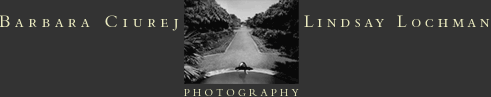L A N D S C A P E SP O R T F O L I O S:P L A Y T I M ES H I N O L AE G G S I S T E N T I A L   D I L E M M AD E A T H   T A K E S   A   H O L I D A YR E A P   J U S T   W H A T   Y O U   S O WT H E   J O U R N E YL A N G U I D   V E R T I G OF E R T I L E   G I R D L Ehome  l  artists’ statement  l  contact us